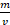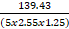# Given dry operating mass 5210 zero fuel mass

Classified in Chemistry

Written at onEnglish with a size of 5.51 KB.

 The formula of hydrated calcium chloride is CaCl2.XH2O. Calculate X if the mass of dry salt is 11.1g and the mass of water in the compound is 3.6g. First get the mole ratios of CaCl2 and H2OThen divide the ratios by the smallest member to get single integer11.1/110 :  3.6/180.1 : 0.2   or 1 : 2CaCl2 . 2H2O

 A 10ml sample of alcohol is pipetted into a flask with stopper. The mass is found by difference to be 7.899g. Calculate the density of the liquid. Density D == 7.899/10 = 0.8g/ml.One digit. The mass of an unknown rectangular block is 139.43g. If the block measures 5.00cm by 2.55cm by 1.25cm, what is its density? Density D === 8.75g/cm3 What is the meaning of the word sublimation. Solid changing directly into gas without changing to liquid first.